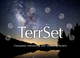Hi,

I really like the Earth Trends Modeler and its Series Trend Analysis! Normally, I use Mann-Kendall trend and the Mann-Kendall significance. Now I want to try linear correlation - but there is no significance function. Can I use Mann-Kendall significance also for testing linear correlation? Or is there another function to calculate significance of linear correlation?

Thank you so much for an answer!

Best, Julia

•Clark Labs

Hi Julia,

In general, we recommend the use of the Theil-Sen measure as an estimate of trend. The Mann-Kendall significance test is appropriate in this context. If you wish to test the linear correlation, a standard test of correlation (for which the series length, n, is the critical parameter) can be used. You can simply reclassify the image based on the confidence interval associated with the test. However, with either technique, serial correlation is a problem (the tendency of anomalies at any point in time to be similar to those at nearby points in time). There is a simple way to handle this in ETM. Go to the PREPROCESS panel and the DETREND/PREWHITEN panel. First, use the Durbin-Watson option to test each pixel for serial correlation. Then run the Trend Preserving Prewhitening option to remove the serial correlation. Then estimate the trend or correlation and apply the significance test.

Ron Eastman

Clark Labs

•